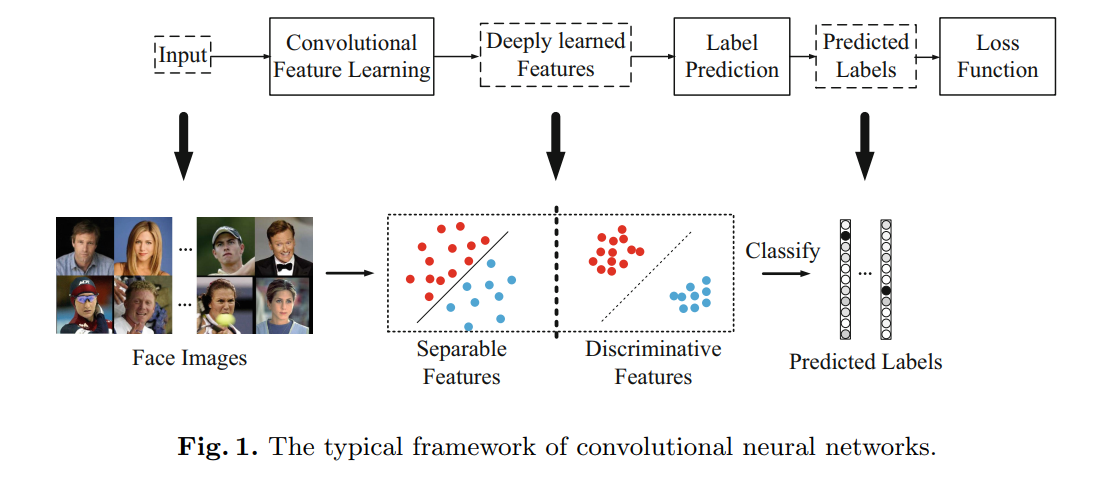[ECCV 2016] A Discriminative Feature Learning Approach for Deep Face Recognition

ECCV 2016的这篇文章主要是提出了一个新的Loss：Center Loss，用以辅助Softmax Loss进行人脸的训练，主要目的是利用softmax loss来分开不同类别，利用center loss来压缩同一类别，最终获取discriminative features. 本文主要对center loss的一些细节做分析介绍，若对论文实验感兴趣，请自行看作者原文。

## [center loss]

center loss意思即为：为每一个类别提供一个类别中心，最小化min-batch中每个样本与对应类别中心的距离，这样就可以达到缩小类内距离的目的。就是每个这个batch中每个样本对应的类别中心，它和特征x的维度一样，对相关变量求导如下：

## [center 的计算与更新]

In each iteration, the centers are computed by averaging the features of the corresponding classes

layer {
name: "center_loss"
type: "CenterLoss"
bottom: "fc5"
bottom: "label"
top: "center_loss"
param {
lr_mult: 1
decay_mult: 2
}
center_loss_param {
num_output: 10572
center_filler {
type: "xavier"
}
}
loss_weight: 0.008
}


if (this->blobs_.size() > 0) {
LOG(INFO) << "Skipping parameter initialization";
} else {
//每个类别都有自己的一个类别中心，每个类别的特征是K_维
//故center_shape为[N_, K_]
this->blobs_.resize(1);
// Intialize the weight
vector<int> center_shape(2);
center_shape = N_;
center_shape = K_;
this->blobs_.reset(new Blob<Dtype>(center_shape));
// fill the weights
// 初始化center_shape的值
shared_ptr<Filler<Dtype> > center_filler(GetFiller<Dtype>(
this->layer_param_.center_loss_param().center_filler()));
center_filler->Fill(this->blobs_.get());

}  // parameter initialization


## [center 的“梯度”具体是怎么得到的]

caffe源码如下：

// Gradient with respect to centers
// 计算center反向传播的更新量，
if (this->param_propagate_down_) {
const Dtype* label = bottom->cpu_data();
Dtype* center_diff = this->blobs_->mutable_cpu_diff();//存center更新值的地方
//variation_sum_data数据维度和center一样，用于更新center时候的计算，不出现的类别都为0
Dtype* variation_sum_data = variation_sum_.mutable_cpu_data();
const Dtype* distance_data = distance_.cpu_data();

// \sum_{y_i==j}
// 计算一个batch中出现的样本的类别的更新量
caffe_set(N_ * K_, (Dtype)0., variation_sum_.mutable_cpu_data());
for (int n = 0; n < N_; n++) {    // N个类别，每个都要试一遍，时间复杂度O(N_*M_)
int count = 0;                  //当前类别出现的个数
for (int m = 0; m < M_; m++) {  //m个样本
const int label_value = static_cast<int>(label[m]); //当前样本的类别id
if (label_value == n) {
count++;
//variation_sum_data初始值为0, 0-distance_data,即为公式中的相减操作
caffe_sub(K_, variation_sum_data + n * K_, distance_data + m * K_, variation_sum_data + n * K_);
}
}
//center_diff = (Dtype)1./(count + (Dtype)1.) * variation_sum_data
//K_为个数，特征的维度，即计算的偏移量是一个向量
caffe_axpy(K_, (Dtype)1./(count + (Dtype)1.), variation_sum_data + n * K_, center_diff + n * K_);
}
}

## [疑点]

--------更新线---------